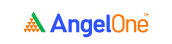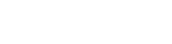# The density curveRemember we plotted the graphs for the differential, the spread and the price ratio for TCS and Infosys shares for a one-month period in chapter two of this module? Now, while plotting the data points for these variables can tell you how they’ve increased or decreased over a given period, they cannot really tell you much about how much these variables have deviated from their normal range.

That’s the standard deviation’s job, as we’ve seen in the previous chapter. That’s the statistical tool that tells you how the data points in a set are distributed. Now, you know we have three variables that can help judge the correlation between two stocks, the question that crops up is how do you know which variable to select?

### Differential, spread and price ratio: Which one should you choose?

The short answer is that you can choose any one of these. There’s no hard and fast rule here. But, let’s back up a bit and see what these three variables represent.

• The differential is the difference in the closing prices of the stocks.
• The spread is the difference in the daily change in the stock’s closing prices.
• The price ratio is the closing price of one stock divided by the closing price of the other.

Now, the first two variables - the differential and the spread - are both slightly less relative or comparative than the third variable. This is because even if two stocks are highly correlated, there’s no guarantee that their prices have to move by the same extent. Sure, they may move in the same direction, but not by the same measure.

The ratio, on the other hand, gives you a more comparative assessment of the prices of the two stocks. So, for the sake of this discussion, let’s make use of the price ratio to check out how to identify pair trading opportunities. But of course, you could always choose to make use of either of the other two variables. It’s entirely up to you.

### Identifying the trigger for a pair trade

All the three variables we’ve seen are calculated based on the closing prices of the stocks, right? So, given how the closing prices change every trading day, it’s logical to conclude that the differential, the spread and the price ratio also all change on a day-to-day basis, isn’t it?

So, in the 1-year data set that we’ve been using, you’ll recall that we have around 249 data points for the closing price of the TCS share and the Infosys share (from 17 Feb, 2020 to 15, Feb 2021). This means we’ll have around as many data points for the differential, the spread and the price ratio of these stocks.

In the previous chapter, we’ve calculated the basic statistical metrics for these three variables. Let’s take a quick look at these details here.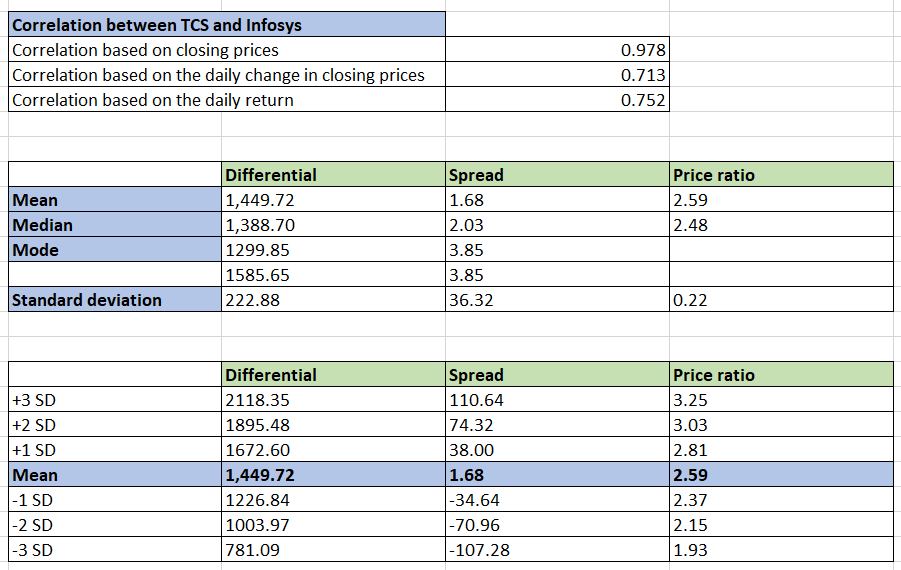Now, each of the three variables has a mean, doesn't it? Let’s plot the data points for the differential, the spread and the price ratio and see how they move upward or downward on a day-to-day basis, compared to the average.

### The differential chart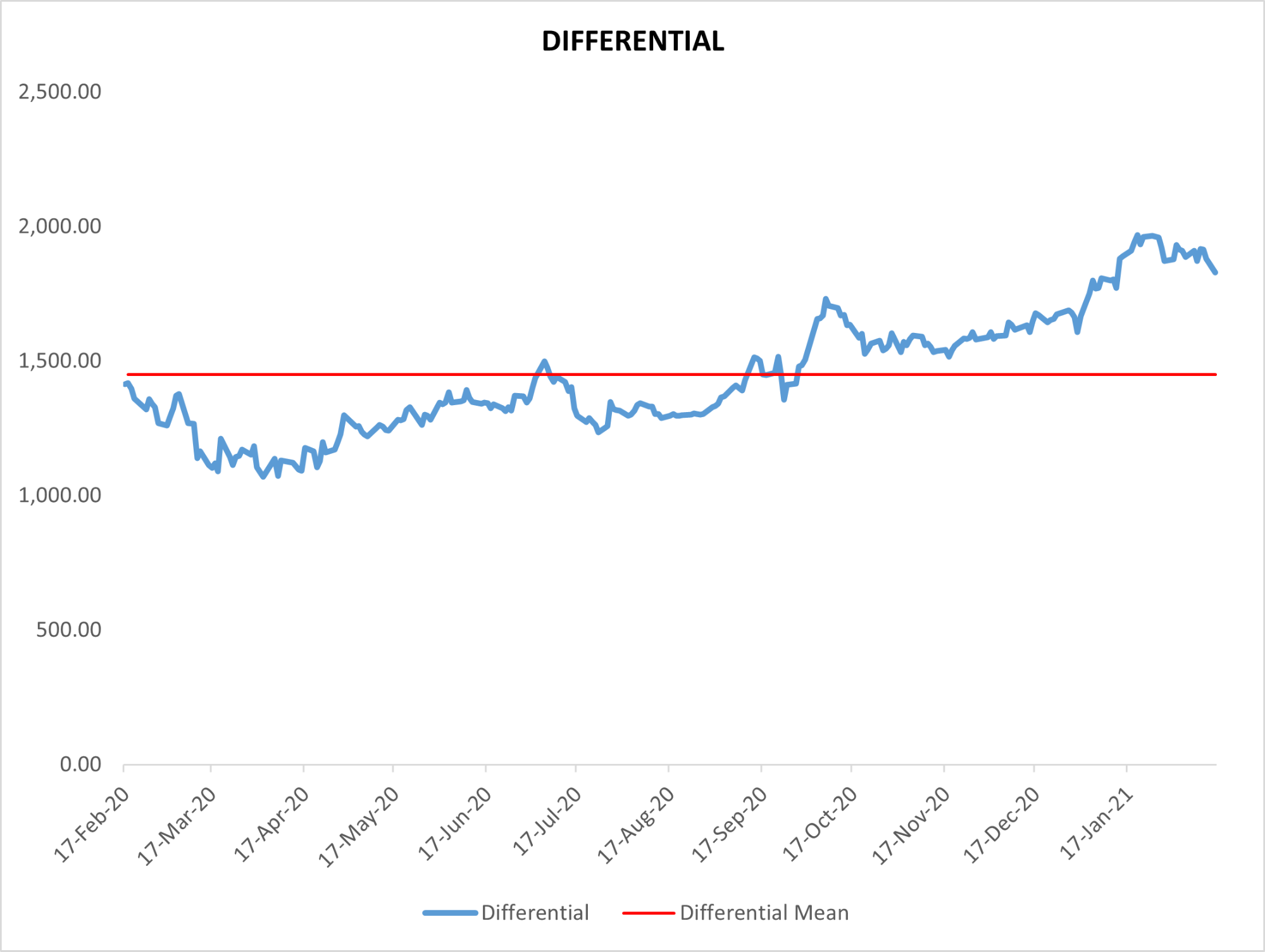The red line shown represents the mean value for the differential (1449.72). You’ll notice that the curve moves above and below the mean, but eventually remains more or less centred around it.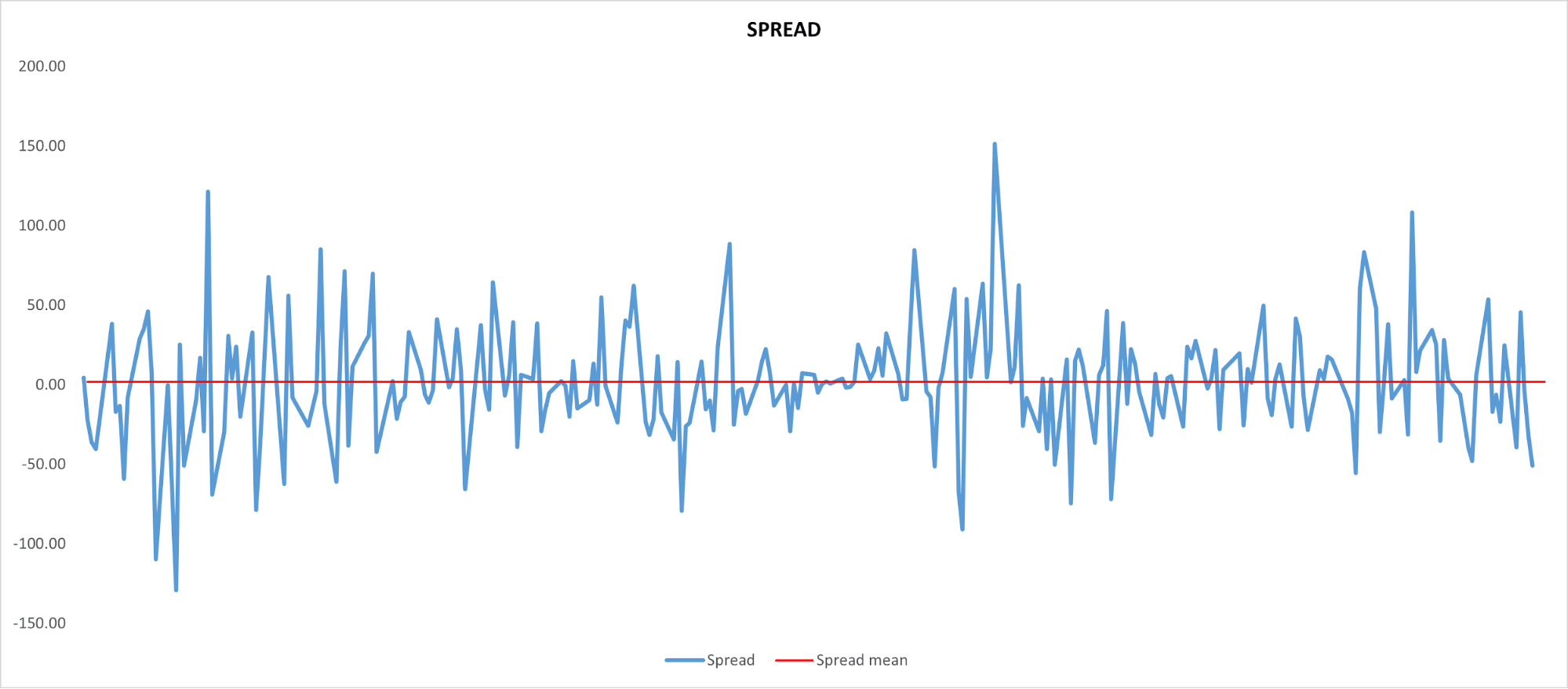Here too, the red line represents the mean spread (1.68). The variations of the spread are much more intense, but again, as with all data points, the values remain centred around their arithmetic mean, always returning to nearbout the average despite the steep peaks and troughs.

### The price ratio chart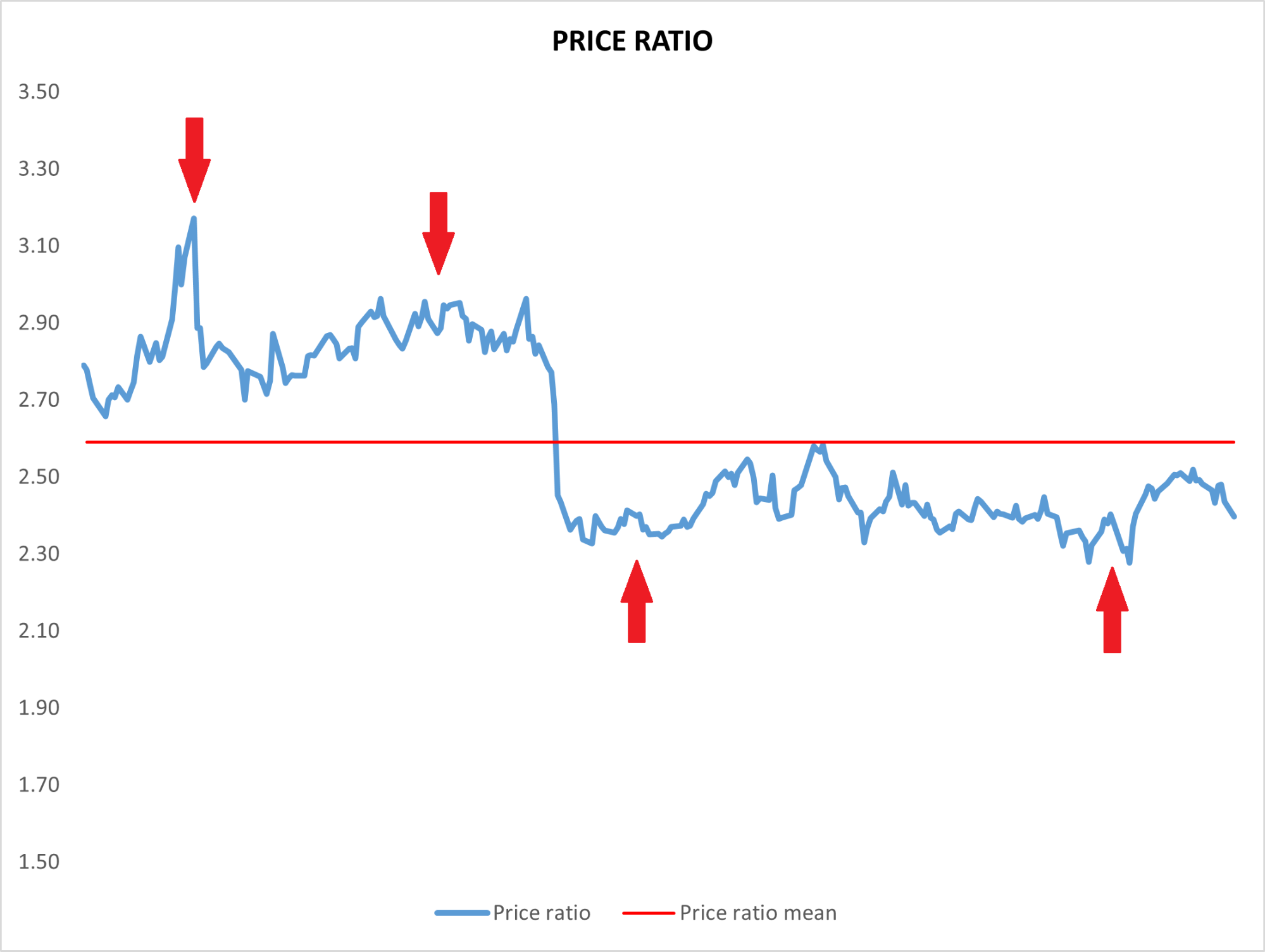Now, this is the chart you’ll want to pay extra attention to, since for the sake of discussion, we’ll stick with the price ratio as the selected variable. The red line represents the mean price ratio (2.59). Over the 1-year period, the price ratio for the closing prices of TCS and Infosys shares move upward and downward frequently, with respect to the mean. But it always tends to go back to values that are centred around the mean.

See the points where there are red arrows marked on the chart? The first two red arrows represent peaks. Despite these peaks, the ratio has eventually reverted to the mean region. Similarly, the last two arrows - they represent troughs. Eventually, the ratio rises back up to nearabout the mean, after attaining troughs.

So, what does this tell us?

• When the ratio peaks, it’s likely that it will eventually drop and revert to nearabout the mean
• When the ratio drops, it’s likely that it will eventually rise and revert to nearabou the mean

This gives you a sense of what may happen in the near future. So, that’s how a trading opportunity arises. As a general rule of thumb, here’s the broad logic behind the ratio movement.

• When the ratio is well above the mean, you expect it to fall. This means you will take a short position in the ratio.
• When the ratio is well below the mean, you expect it to rise. This means you will take a long position in the ratio.

But wait a minute. How can you take a position in the ratio? You can only do that with stocks, isn’t it?

Well, that’s correct. But here, we’re dealing with a pair of stocks. So, the exact kind of positions you take in them - we’ll see that in the next chapter. For now, let’s just understand how to read the ratio movements and identify trigger points for a pair trade.

With regard to the movement of the price ratio, you’ve seen that it’s likely to always revert to the mean. But how likely is it? Does the probability of mean reversion vary across different points on the curve? It turns out they do.

And again, it’s statistics to the rescue. There’s one last statistical metric we’ll need to see before we get around to setting up a pair trade - and that metric is the density curve. Once again, we can simply make use of an excel function to calculate this.

### What is the density curve?

The density curve represents the probability of a variable reverting to the mean. That’s why it’s also sometimes referred to as the probability density curve. In the price ratio chart we saw above, you’ll recall that some points were higher than average, while some were lower. Let’s take up the points where the ratio was above the average by a significant amount.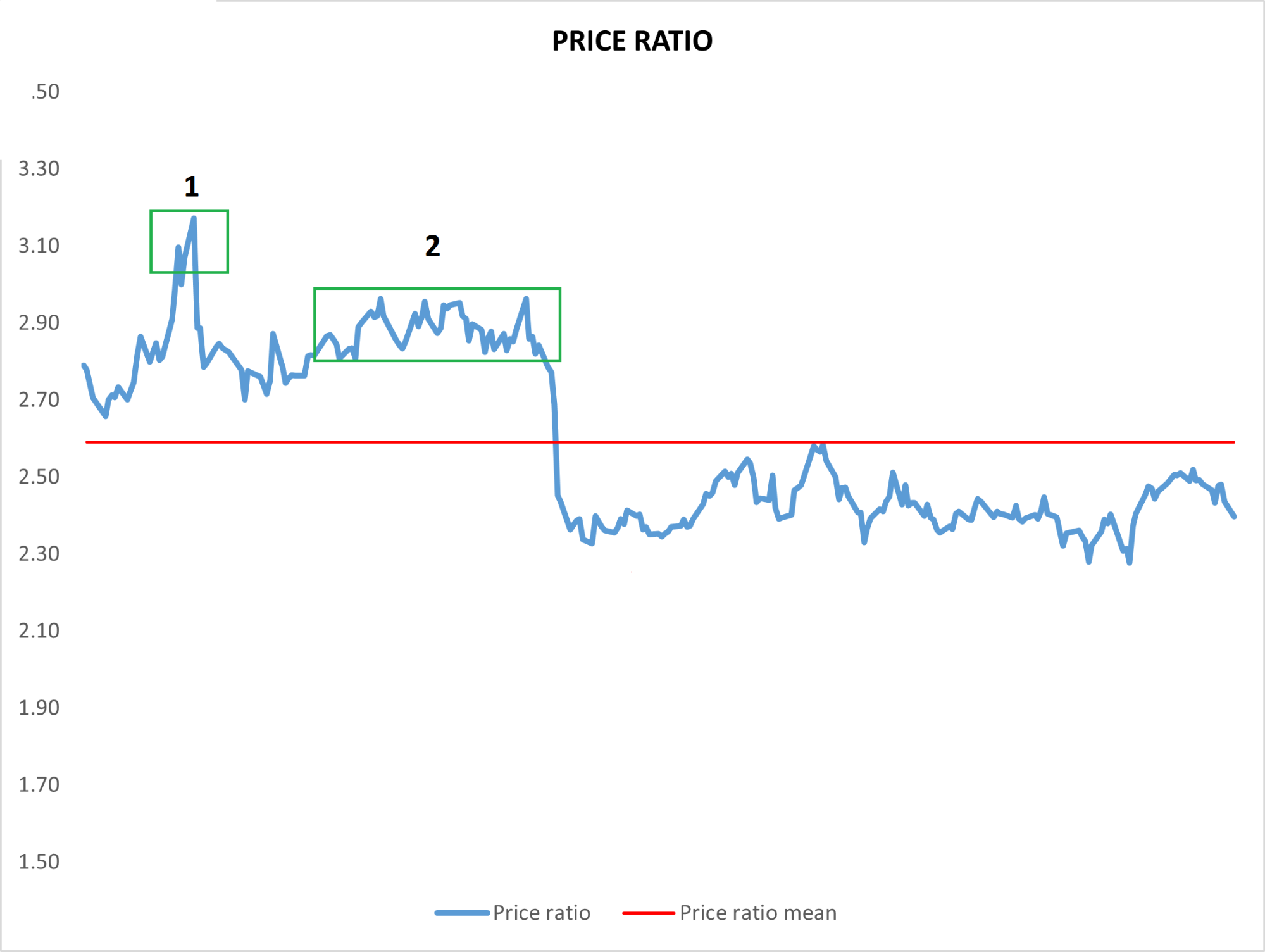See the two areas marked as 1 and 2? Those are the two main peaks we can identify. Now, how do you know at which peak it’s more logical to initiate a pair trade? Well, since you will initiate the trade based on the assumption that the ratio will revert to the mean, it’s only sensible to choose the peak where the probability of mean reversion is higher.

And how do you identify that probability? That’s where the probability density curve comes in.

While the density curve does give you more accurate results, let’s see what common statistical logic suggests. Do you recall the empirical rule?

According to this rule:

• 68% of values are within one standard deviation (1SD) away from the mean.
• 95% of values are within two standard deviations (2SD) away from the mean.
• 99.7% of values are within three standard deviations (3SD) away from the mean.

So, in the context of the price ratio, here’s what it means:

• If the ratio is in the 1SD zone, it has a 68% chance of mean reversion.
• If the ratio is in the 2SD zone, it has a 95% chance of mean reversion.
• If the ratio is in the 3SD zone, it has a 99.7% chance of mean reversion.

Simply put, the further the ratio deviates from the mean, the higher the chances of it reverting to the mean. So, the higher the standard deviation, the greater the probability of the ratio falling back down (or rising back up) to get closer to the mean.

The density curve represents this relationship beautifully. The normal distribution function in excel can be used to quickly calculate the density curve for the price ratio of TCS and Infosys stocks over the 1-year period we took into consideration.

Here’s a preview of how it’s done for the first few values.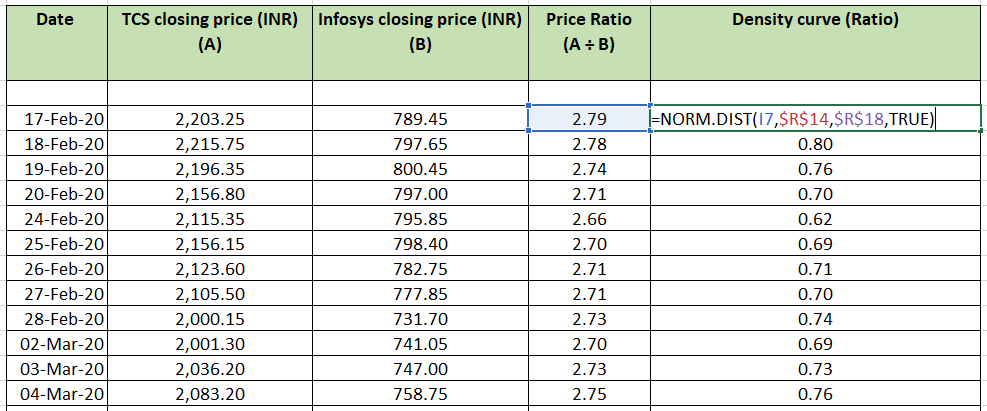To explain further, the function is filled in as:

NORM.DIST(price ratio value, price ratio average, price ratio’s SD, TRUE)

The TRUE here refers to the fact that we’re using the cumulative distribution function. FALSE suggests that the formula will use the probability mass function, which we’re not going with here. So, for simplicity’s sake, it’s best to type in TRUE in the last field of the function.

A quick note for statistic-lovers:

• Since the density curve is essentially the probability of the price ratio (or any other variable) reverting to the mean, it always lies between 0 and 1.
• The higher the standard deviation for a price ratio, the greater its density curve is. In other words, the closer it is to 1.

#### Wrapping up

And bingo! You now have the last statistical tool needed to locate pair trade trigger points. The final piece of the puzzle is the density curve. And what a journey it has been, from the three variables, to mean, media, and mode, to the standard deviation, and finally, to the density curve.

Without further ado, head to the next chapter to see how all of these concepts come together to help pair traders.

#### A quick recap

• You can make use of any of the three variables to identify the trigger point for the pair trade.
• When you plot the variables on a graph, you can see how they deviate from their mean value.
• But no matter how deep or high the deviation is, the variable generally tends to revert to the mean.
• It’s this awareness that serves as the basis for a pair trade.
• The greater the standard deviation is - or the more a variable deviates from the mean - the higher the chances of it reverting.
• The probability of mean reversion is calculated using the density curve.Take the quiz for this chapter & mark it complete.

How would you rate this chapter?

Get Information Mindfulness!

Catch-up With Market

News in 60 Seconds.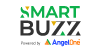The perfect starter to begin and stay tuned with your learning journey anytime and anywhere.